# CPS100 • INTRODUCTION TO COMPUTERS

## SUMMER 2020 Semester • LAKELAND UNIVERSITY

Formulas

A formula is basically a calculation. A spreadsheet really is, a giant, special calculator.

Formulas can be simple or complex. The most basic formulas are simple mathematical equations. For example, 2+2.

Before We Continue: Excel uses operators, or mathematical signs; you should know the vocabulary for these.

 Sign keyboard sign name verb / noun example + + plus add (-ition) 2+3=5 - - minus subtract (-ion) 8-2=6 x * times multiply (-ication) 3*4=12 ÷ / divide divide (division) 15/3=5 (superscript) ^ power to the power of/nth power 3^2 = = equal equals 2+2=4

#### Simon Says "Equal"!

Have you ever played a game called "Simon Says"? In this game, a leader gives commands to everyone who is playing. Everyone must follow the leader's commands (for example, "raise your right hand," or "jump up and down"). However, the players must follow the command only if the leader begins the command with the words, "Simon Says." If the leader gives a command without those words and a player follows the command, that player must leave the game.

In Excel, the equal sign = is like "Simon Says" for formulas. A formula must begin with an equal sign. If there is no equal sign at the beginning of the command, then Excel will ignore it. But if you put the = first, then Excel will see it as a formula and it will calculate and show the answer.

For example, if you type"2+2=" into a cell and hit the "enter" or "tab" key, Excel will not change what you typed. However, if you type"=2+2" into a cell and hit the "enter" or "tab" key, Excel show you the answer, "4."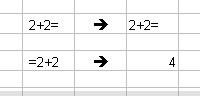Note that when you type text, it stay on the left side of the cell. However, when you type a formula or even any number and hit "tab" or "enter," the number goes to the right.

#### Layers

There are two "layers" to a spreadsheet. The "top" layer is the one you see, and it has all the text and numbers. The "bottom" layer is hidden "below" the top layer, and it holds all the formulas.

If you type a formula (for example, =6*4) and hit "tab" or "enter," the formula will disappear and the answer to the formula (24) will appear in the cell. Has the formula vanished? Is it gone, destroyed, lost? No--it is still there, on the bottom layer, the formula layer. The answer is "above" it, hiding the formula below.

You can see the formula below in two ways: first, you can select the cell with the formula, and then look at the formula bar at the top of the spreadsheet. There is a bold "equal" sign at the left of the formula bar. The formula bar always shows the formula in any cell you select.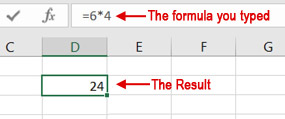The second way to see a formula is to double-click on the cell which hides the formula.

Think of this as Excel having two layers, one which you can see (the top "data" layer), and one below which you cannot see (the bottom "formula" layer). The formula layer only becomes visible when you look in the Formula Bar or when you double-click on the cell.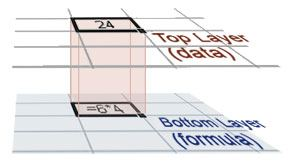#### References

For a spreadsheet program to really use its power, though, you have to do more than just put plain numbers in calculations. You have to use references. These allow the feature of automatic recalculation to be used.

A reference is when you use a cell or range address (for example, "B2" or "B2:B10") inside a formula. For example:

= B2+B3

The above formula will go to the cells B2 and B3, retrieve their values, and then perform the calculation.

When you do that, we do not call B2 or B3 an "address" any more. Instead, it is called a "reference." One cell address inside a formula is called a cell reference, and a range of cell addresses inside a formula is called a range reference.

A reference is just that: it refers to (points to) the value in the cell of that address. If you type "24" into the cell B3, then B3=24. If you change the number in B3, the reference changes.

References are powerful because they allow you to very easily change any formula that refers to the cell. For example, if you type a formula with numbers, you have to type something like this:

=22+34+17+91+47

Now, look at the example below: the numbers are entered in cells B2 to B6; the formula is entered into B8. However, notice that the formula is simple a calculation of the numbers listed above. There is no connection between the numbers in B2 to B6 and the numbers in the formula: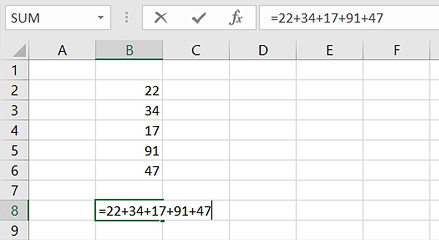Note that if one of the numbers in the cells above is changed, it has no effect on the formula (which shows the result in B8, and the formula is listed in the Formula Bar) because the number in B4 is not referred to in the formula in B8: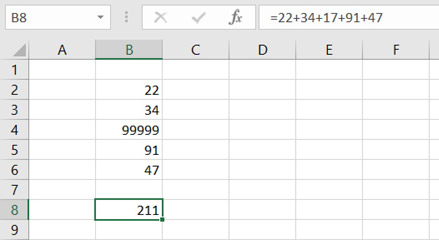Instead, if we use references, the formula will be referring directly to each cell—in the example, below, it is adding what is in each cell (B2+B3+B4+B5+B6).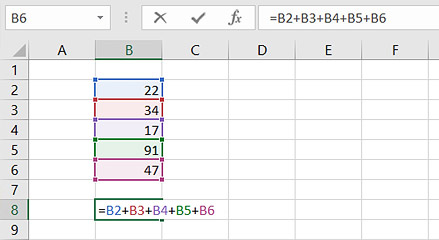Because the formula is referring directly to each cell, if you change the number in any one cell, it will change the result in the formula as well: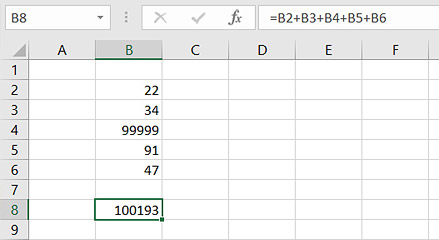That's what makes Excel really powerful: when you want to make a change to any number, the corrections are automatically made in all formulas that refer to that cell. Let's say the number "22" in B2 is wrong; it should be "23". Just type the new number into B2, and the result in B8 will change automatically. Again, this is the "automatic recalculation" that was introduced above.

In other words, each formula doesn't have the numbers inside of it; instead, the formula looks at cells which have the numbers inside of them. Change a cell's number, and all calculations connected to that cell by references will change immediately.

### Terms to Know

formulaa statement for a calculation
operatora mathematical sign or symbol for a calculation
formula barthe bar near the top of the Excel window which shows the formula for a cell
referenceusing the address of a cell or a range in a calculation
cell referencea cell address used in a formula
range referencea range address used in a formula
automatic recalculationthe ability of a spreadsheet app to automatically update the results of all connected calculations if any data in the cells are changed

Previous Chapter Chapter Practice Next Chapter